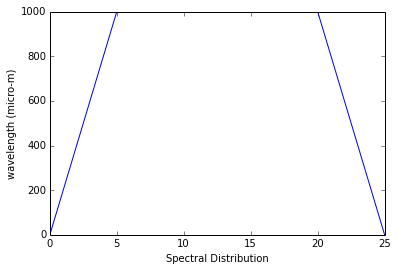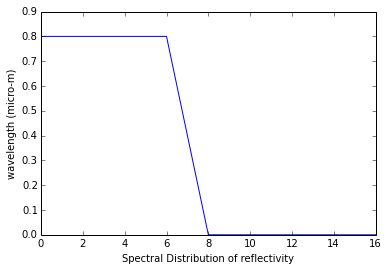Example 12.1 Page 731¶

In :
import math

A1 = .001		;#[m^2] Area of emitter
In  = 7000		;#[W/m^2.Sr] Intensity of radiation in normal direction
A2 = .001		;#[m^2] Area of other intercepting plates
A3 = A2			;#[m^2] Area of other intercepting plates
A4 = A2			;#[m^2] Area of other intercepting plates
r = .5			;#[m] Distance of each plate from emitter
theta1 = 60.	;#[deg] Angle between surface 1 normal & direction of radiation to surface 2
theta2 = 30.	;#[deg] Angle between surface 2 normal & direction of radiation to surface 1
theta3 = 45.	;#[deg] Angle between surface 1 normal & direction of radiation to surface 4
#calculations

#From equation 12.2
w31 = A3/(r*r);
w41 = w31;
w21 = A2*math.cos(theta2*0.0174532925)/(r*r);

#From equation 12.6
q12 = In*A1*math.cos(theta1*0.0174532925)*w21;
q13 = In*A1*math.cos(0*math.pi/180.)*w31;
q14 = In*A1*math.cos(theta3*0.0174532925)*w41;
#results

print '%s %d %s' %("\n (a) As Intensity of emitted radiation is independent of direction, for each of the three directions I = ",In,"W/m^2.sr")
print '%s' %("\n\n (b) By the Three Surfaces\n         Solid angles subtended                Rate at which radiation is intercepted \n")
print '%s %.2e %s' %("w4-1 =",w41," sr")
print '%s %.2e %s' %("\t \t \t \t  \t \t q1-4 =",q14," W")
print '%s %.2e %s' %("\nw3-1 = ",w31," sr")
print '%s %.2e %s' %("\t \t \t \t \t \t q1-3 =",q13," W")
print '%s %.2e %s' %("\n w2-1 = ",w21," sr")
print '%s %.2e %s' %("\t \t \t \t \t \tq1-2 = ",q12," W ");
#END
(a) As Intensity of emitted radiation is independent of direction, for each of the three directions I =  7000 W/m^2.sr

(b) By the Three Surfaces
Solid angles subtended                Rate at which radiation is intercepted

w4-1 = 4.00e-03  sr
q1-4 = 1.98e-02  W

w3-1 =  4.00e-03  sr
q1-3 = 2.80e-02  W

w2-1 =  3.46e-03  sr
q1-2 =  1.21e-02  W

Example 12.2 Page 734¶

In :
import math
import matplotlib.pyplot as plt
%matplotlib inline
#calculations

x=([0, 5, 20, 25]);
y=([0, 1000, 1000, 0]);

plt.plot(x,y);
plt.xlabel("Spectral Distribution")
plt.ylabel("wavelength (micro-m)")
#By Equation 12.4
G = 1000*(5-0)/2. +1000*(20-5)+1000*(25-20)/2.;
#results

print '%s %d %s' %("\n G =",G," W/m^2");
#END
Populating the interactive namespace from numpy and matplotlib

G = 20000  W/m^2Example 12.3 Page 741¶

In :
import math

T = 2000.								;#[K] temperature of surface
stfncnstt = 5.67*math.pow(10,-8)		;#[W/m^2.K^4] Stefan-Boltzmann constant
E = stfncnstt*T*T*T*T;        			#[W/m^2]
#calculations

#From Table 12.1
constt1 = 2195.    ;   					#[micro-m.K]
wl1 = constt1/T;
#From Table 12.1
constt2 = 9382.   ;    					#[micro-m.K]
wl2 = constt2/T;

#From Weins Law, wlmax*T = consttmax = 2898 micro-m.K
consttmax = 2898         				;#micro-m.K
wlmax = consttmax/T;
#from Table 12.1 at wlmax = 1.45 micro-m.K and T = 2000 K
I = .722*stfncnstt*T*T*T*T*T/10000.;
Eb = math.pi*I;

G = E;        #[W/m^2] Irradiation of any small object inside the enclosure is equal to emission from blackbody at enclosure temperature
#results

print '%s %.2e %s' %("\n (a) Spectral Emissive Power of a small aperture on the enclosure =",E," W/m^2.Sr for each of the three directions")
print '%s %.1f %s' %("\n (b) Wavelength below which 10percent of the radiation is concentrated = ",wl1," micro-m \n")
print '%s %.2f %s' %(" Wavelength above which 10percent of the radiation is concentrated = ",wl2," micro-m \n")
print '%s %.2e %s %.2e %s' %("(c) Spectral emissive power and wavelength associated with maximum emission is ",Eb,"micro-m and",wlmax," W/m^2.micro-m respectively")
print '%s %.2e %s' %("\n (d) Irradiation on a small object inside the enclosure =",G,"W/m^2");
#END
(a) Spectral Emissive Power of a small aperture on the enclosure = 9.07e+05  W/m^2.Sr for each of the three directions

(b) Wavelength below which 10percent of the radiation is concentrated =  1.1  micro-m

Wavelength above which 10percent of the radiation is concentrated =  4.69  micro-m

(c) Spectral emissive power and wavelength associated with maximum emission is  4.12e+05 micro-m and 1.45e+00  W/m^2.micro-m respectively

(d) Irradiation on a small object inside the enclosure = 9.07e+05 W/m^2

Example 12.4 Page 743¶

In :
import math
import scipy
from scipy import integrate

T = 1500.								;#[K] temperature of surface
stfncnstt = 5.67*math.pow(10,-8)		;#[W/m^2.K^4] Stefan-Boltzmann constant
#calculations

#From Equation 12.26 Black Body Radiation
Eb = stfncnstt*T*T*T*T;        			#[W/m^2]

#From Table 12.1 as wl1*T = 2*1500 (micro-m.K)
F02 = .273;
#From Table 12.1 as wl2*T = 4*1500 (micro-m.K)
F04 = .738;
def func(x):
func = 2*math.cos(x) *math.sin(x)
return func;

#From equation 12.10 and 12.11
delE = i1 *(F04-F02)*Eb;
#results

print '%s %.2e %s' %("\n Rate of emission per unit area over all directions between 0 degC and 60 degC and over all wavelengths between wavelengths 2 micro-m and 4 micro-m =",delE," W/m^2");
#END
Rate of emission per unit area over all directions between 0 degC and 60 degC and over all wavelengths between wavelengths 2 micro-m and 4 micro-m = 1.00e+05  W/m^2

Example 12.5 Page 748¶

In :
import math

T = 1600.								;#[K] temperature of surface
wl1 = 2            						;#[micro-m] wavelength 1
wl2 = 5            						;#[micro-m] wavelength 2
stfncnstt = 5.67*math.pow(10,-8);		#[W/m^2.K^4] Stefan-Boltzmann constant
# From the given graph of emissivities
e1 = .4;
e2 = .8;
#calculations

#From Equation 12.26 Black Body Radiation
Eb = stfncnstt*T*T*T*T; 	       		#[W/m^2]

#Solution (A)
#From Table 12.1 as wl1*T = 2*1600 (micro-m.K)
F02 = .318;
#From Table 12.1 as wl2*T = 5*1600 (micro-m.K)
F05 = .856;
#From Equation 12.36
e = e1*F02 + e2*(F05 - F02);

#Solution (B)
#From equation 12.35
E = e*Eb;

#Solution (C)
#For maximum condition Using Weins Law
consttmax = 2898.        				;#[micro-m.K]
wlmax = consttmax/T;

#equation 12.32 with Table 12.1
E1 = math.pi*e1*.722*stfncnstt*T*T*T*T*T/10000.;

E2 = math.pi*e2*.706*stfncnstt*T*T*T*T*T/10000.;
#results

print '%s %.3f' %("\n (a) Total hemispherical emissivity =",e)
print '%s %d %s' %("\n (b) Total emissive Power =",E/1000. ," kW/m^2")
print '%s %.1f %s %.1f %s ' %("\n (c) Emissive Power at wavelength 2micro-m is greater than Emissive power at maximum wavelength \n     i.e.",E2/1000.," kW/m^2 >",E1/1000.," kW/m^2")
print '%s %d %s' %("\n     Thus, Peak emission occurs at",wl1,"micro-m");
#END
(a) Total hemispherical emissivity = 0.558

(b) Total emissive Power = 207  kW/m^2

(c) Emissive Power at wavelength 2micro-m is greater than Emissive power at maximum wavelength
i.e. 105.5  kW/m^2 > 53.9  kW/m^2

Thus, Peak emission occurs at 2 micro-m

Example 12.6 Page 751¶

In :
import math
import scipy
from scipy import integrate

T = 2000.								;#[K] temperature of surface
wl = 1            						;#[micro-m] wavelength
stfncnstt = 5.67*math.pow(10,-8);		#[W/m^2.K^4] Stefan-Boltzmann constant

# From the given graph of emissivities
e1 = .3;
e2 = .6;
#calculations

#From Equation 12.26 Black Body Radiation
Eb = stfncnstt*T*T*T*T;        						#[W/m^2]
def func1(x):
func1=e1*math.cos(x) *math.sin(x);
return func1;

def func2(x):
func2=e2*math.cos(x) *math.sin(x);
return func2;

#Equation 12.34
e = 2*(i1+i2);

# From Table 12.1 at wl = 1 micro-m and T = 2000 K.

I = .493*math.pow(10,-4) * stfncnstt*T*T*T*T*T        ;#[W/m^2.micro-m.sr]

In = e1*I;

#Using Equation 12.32 for wl = 1 micro-m and T = 2000 K
E = e*math.pi*I;
#results

print '%s %.1f' %('\n Spectral Normal emissivity en =',e1)
print '%s %.2f' %('and spectral hemispherical emissivity e = ',e)
print '%s %.2e %s' %('\n Spectral normal intensity In =',In,' W/m^2.micro-m.sr')
print '%s %.1e %s' % ('and Spectral emissive power =',E,' W/m^2.micro-m.sr ');
Spectral Normal emissivity en = 0.3
and spectral hemispherical emissivity e =  0.36

Spectral normal intensity In = 2.68e+04  W/m^2.micro-m.sr
and Spectral emissive power = 1.0e+05  W/m^2.micro-m.sr

Example 12.7 Page 759¶

In :
import math
import matplotlib
from matplotlib import pyplot
%matplotlib inline

T = 500.								;#[K] temperature of surface
e = .8;
stfncnstt = 5.67*math.pow(10,-8);		#[W/m^2.K^4] Stefan-Boltzmann constant
#calculations

x=([0, 6, 8, 16]);
y=([.8, .8, 0, 0]);

pyplot.xlabel("Spectral Distribution of reflectivity")
pyplot.ylabel("wavelength (micro-m)");
pyplot.plot(x,y);
pyplot.show();

#From equation 12.43 and 12.44
Gabs = (.2*500/2.*(6.-2.)+500*(.2*(8.-6.)+(1.-.2)*(8.-6.)/2.)+1*500*(12.-8.)+500*(16.-12.)/2.)  ;#[w/m^2]
G = (500*(6.-2.)/2.+500*(12.-6.)+500*(16.-12.)/2.)            									;#[w/m^2]
a = Gabs/G;

#Neglecting convection effects net het flux to the surface
qnet = a*G - e*stfncnstt*T*T*T*T;
#results

print '%s %.2f' %('\n Total, hemispherical absorptivity',a)
print '%s %.2f %s' %('\n Nature of surface temperature change =',qnet,' W/m^2 \n Since qnet > 0, the sirface temperature will increase with the time');
Populating the interactive namespace from numpy and matplotlibTotal, hemispherical absorptivity 0.76

Nature of surface temperature change = 965.00  W/m^2
Since qnet > 0, the sirface temperature will increase with the time

Example 12.8 Page 761¶

In :
import math

T = 5800.								;#[K] temperature of surface
e = .8;
stfncnstt = 5.67*math.pow(10,-8);		#[W/m^2.K^4] Stefan-Boltzmann constant
#calculations and results

#From Table 12.1
#For wl1 = .3 micro-m and T = 5800 K, At wl1*T = 1740 micro-m.K
F0wl1 = .0335;
#For wl1 = .3 micro-m and T = 5800 K, At wl2*T = 14500 micro-m.K
F0wl2 = .9664;

#Hence from equation 12.29
t = .90*(F0wl2 - F0wl1);

print '%s %.2f' %('\n Total emissivity of cover glass to solar radiation =',t);
Total emissivity of cover glass to solar radiation = 0.84

Example 12.9 Page 766¶

In :
import math

Ts = 500.							;#[K] temperature of brick surface
Tc = 2000.           				;#[K] Temperature of coal exposed
stfncnstt = 5.67*math.pow(10,-8)	;#[W/m^2.K^4] Stefan-Boltzmann constant
# From the given graph of emissivities
e1 = .1;    						#between wavelength 0 micro-m- 1.5 micro-m
e2 = .5;    						#between wavelength 1.5 micro-m- 10 micro-m
e3 = .8;    						#greater than wavelength 10 micro-m
#calculations

#From Table 12.1
#For wl1 = 1.5 micro-m and T = 500 K, At wl1*T = 750 micro-m.K
F0wl1 = 0;
#For wl2 = 10 micro-m and T = 500 K, At wl2*T = 5000 micro-m.K
F0wl2 = .634;
#From equation 12.36
e = e1*F0wl1 + e2*F0wl2 + e3*(1-F0wl1-F0wl2);

#Equation 12.26 and 12.35
E = e*stfncnstt*Ts*Ts*Ts*Ts;

#From Table 12.1
#For wl1 = 1.5 micro-m and T = 2000 K, At wl1*T = 3000 micro-m.K
F0wl1c = 0.273;
#For wl2 = 10 micro-m and T = 2000 K, At wl2*T = 20000 micro-m.K
F0wl2c = .986;
ac = e1*F0wl1c + e2*(F0wl2c-F0wl1c) + e3*(1-F0wl2c);
#results

print '%s %.3f' %('\n Total hemispherical emissivity of fire brick wall =',e)
print '%s %d %s' %('\n Total emissive power of brick wall =',E,'W/m^2.')
print '%s %.3f' %('\n Absorptivity of the wall to irradiation from coals =',ac);
Total hemispherical emissivity of fire brick wall = 0.610

Total emissive power of brick wall = 2160 W/m^2.

Absorptivity of the wall to irradiation from coals = 0.395

Example 12.10 Page 768¶

In :
import math

Ts = 300.;							#[K] temperature of surface
Tf = 1200;           				#[K] Temperature of Furnace
stfncnstt = 5.67*math.pow(10,-8);	#[W/m^2.K^4] Stefan-Boltzmann constant
# From the given graph of absorptivities
a1 = .8;    						#between wavelength 0 micro-m- 5 micro-m
a2 = .1;    						#greater than wavelength 5 micro-m
#calculations

#From Table 12.1
#For wl1 = 5 micro-m and T = 1200 K, At wl1*T = 6000 micro-m.K
F0wl1 = 0.738;
#From equation 12.44
a = a1*F0wl1 + a2*(1-F0wl1);
#From Table 12.1
#For wl1 = 5 micro-m and T = 300 K, At wl1*T = 1500 micro-m.K
F0wl1s = 0.014;
#From equation 12.36
e = a1*F0wl1s + a2*(1-F0wl1s);
#results

print' %s %.2f' %('\n For Initial Condition \n Total hemispherical absorptivity = ',a)
print '%s %.2f' %('Emissivity of sphere =',e)
print '%s' %('\n\n Beacuase the spectral characteristics of the coating and the furnace temeprature remain fixed, there is no change in the value of absorptivity with increasing time.')
print '%s %d %s %.2f' %('\n Hence, After a sufficiently long time, Ts = Tf = ',Tf,' K and emissivity equals absorptivity e = a = ',a);

For Initial Condition
Total hemispherical absorptivity =  0.62
Emissivity of sphere = 0.11

Beacuase the spectral characteristics of the coating and the furnace temeprature remain fixed, there is no change in the value of absorptivity with increasing time.

Hence, After a sufficiently long time, Ts = Tf =  1200  K and emissivity equals absorptivity e = a =  0.62

Example 12.11 Page 774¶

In :
import math

Ts = 120+273.;							#[K] temperature of surface
Gs = 750;                 				#[W/m^2] Solar irradiation
Tsky = -10+273.;          				#[K] Temperature of Sky
Tsurr = 30+273.;        	  			#[K] Temperature os surrounding Air
e = .1             		     			;# emissivity
ast = .95                				;# Absorptivity of Surface
asky = e                				;# Absorptivity of Sky
stfncnstt = 5.67*math.pow(10,-8);		#[W/m^2.K^4] Stefan-Boltzmann constant
#calculations

h = 0.22*math.pow((Ts - Tsurr),.3334);	#[W/m^2.K] Convective Heat transfer Coeff
#From equation 12.67
qconv = h*(Ts-Tsurr);        			#[W/m^2]  Convective Heat transfer